maths > construction-high

Construction: Scaling a line, triangle, polygon, circle

what you'll learn...

overview

dividing a line in a given ratio (The method employed is based on properties of similar triangles.)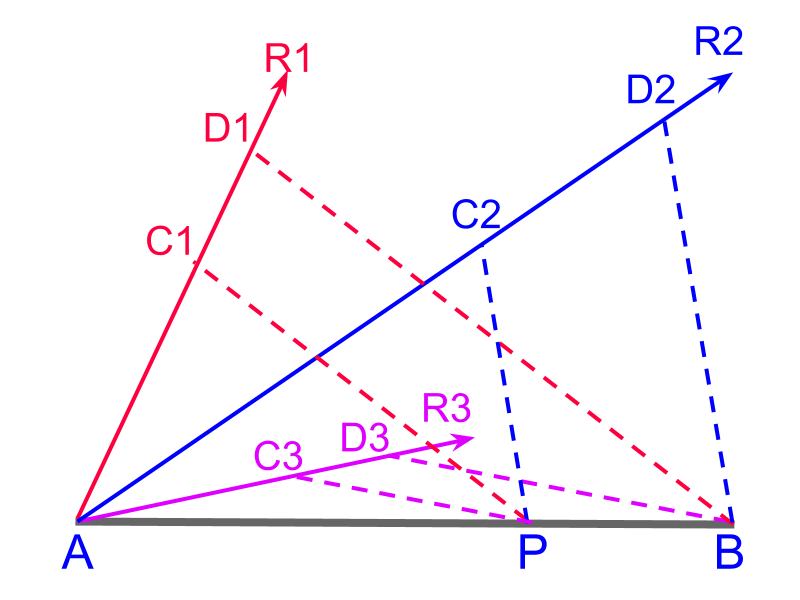scaling or dividing a line in a given ratio (The method employed uses properties of traversal of pair of parallels.)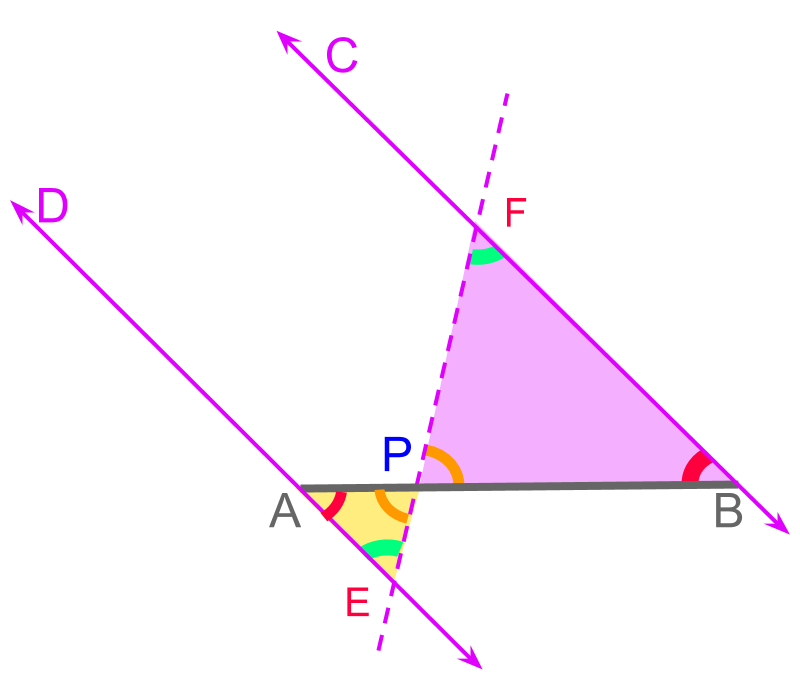scaling a triangle in a given ratio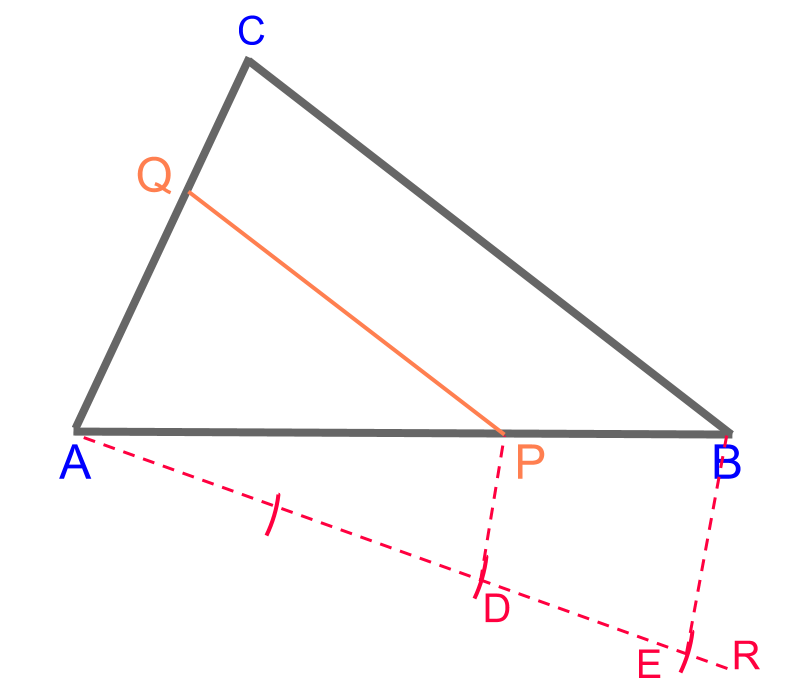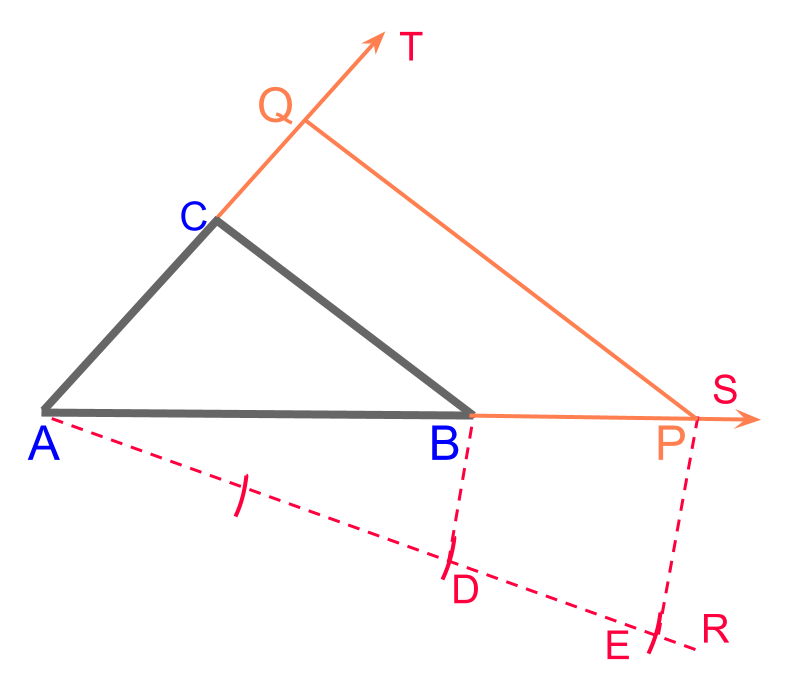scaling a polygon in a given ratio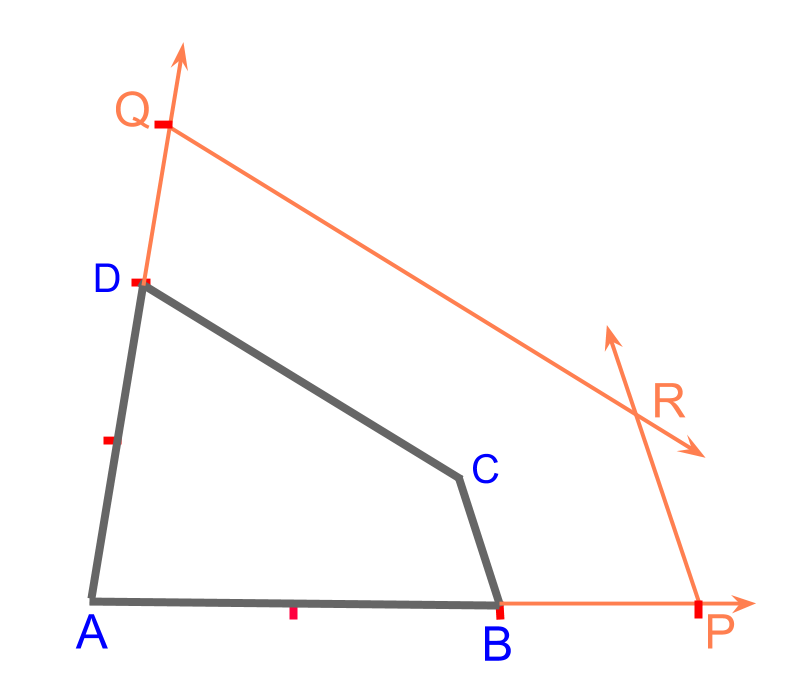scaling a circle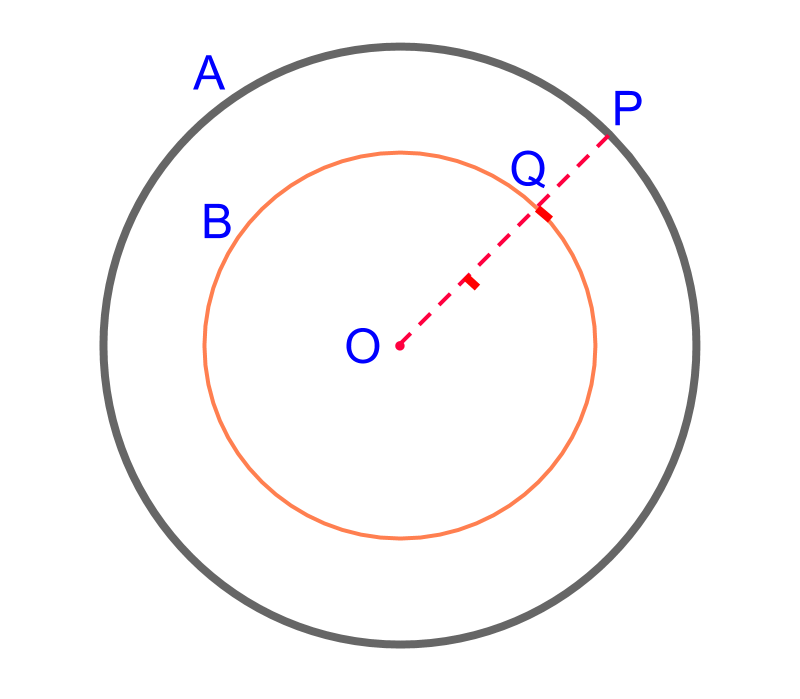problem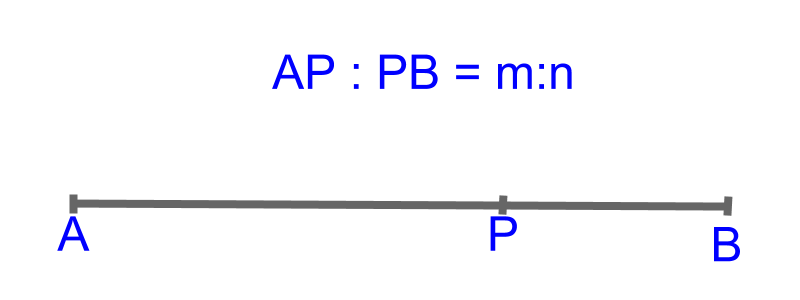Given a line segment $\overline{AB}$$\overline{A B}$, it is required to mark a point $P$$P$ such that, $\overline{AP}:\overline{PB}=m:n$$\overline{A P} : \overline{P B} = m : n$. That is, the $\overline{AB}$$\overline{A B}$ is divided to $\overline{AP}$$\overline{A P}$ and $\overline{PB}$$\overline{P B}$ in $m:n$$m : n$ ratio.

To achieve that, we use properties of similar triangles.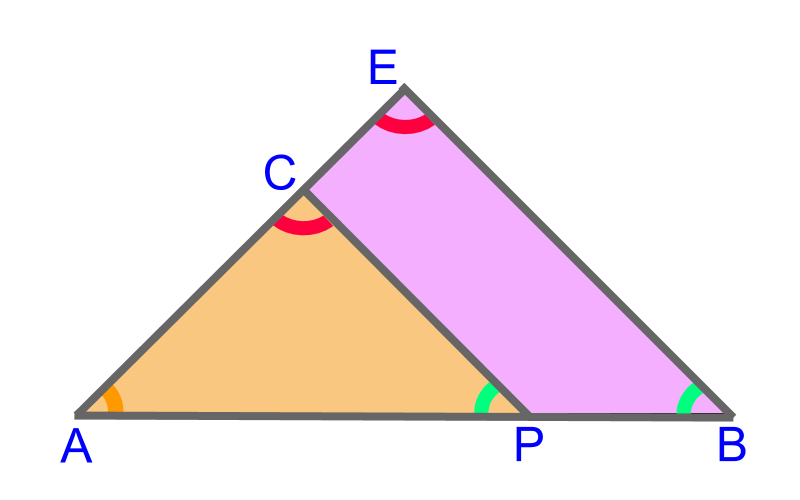A set of similar triangles are formed with $\overline{AB}$$\overline{A B}$ as one of its sides. The formulation is shown in the figure. In such a construction, the following properties are noted.

•  $\overline{AB}:\overline{AP}=\overline{AD}:\overline{AC}$$\overline{A B} : \overline{A P} = \overline{A D} : \overline{A C}$

•  $\overline{EB}\mid \mid \overline{CP}$$\overline{E B} | | \overline{C P}$

The construction is explained in following pages.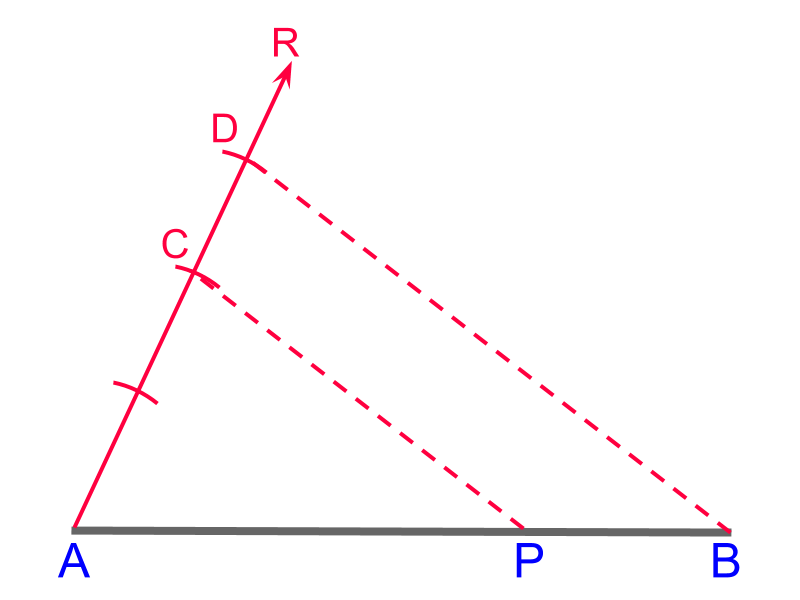The construction is visualized in the following.

•  Construct a ray $\stackrel{\to }{AR}$$\vec{A R}$ at an angle, of any measurement.

•  In a compass, take a measurement

•  Using the compass, mark a number of segments on the ray.

•  Construct line $D$$D$ to $B$$B$

•  Construct a line through the the point $C$$C$

•  The point of intersection of the line through $C$$C$ and $\overline{AB}$$\overline{A B}$ is marked $P$$P$.The figure illustrates $3$$3$ different angles $\angle AR1$$\angle A R 1$, $\angle AR2$$\angle A R 2$, and $\angle AR3$$\angle A R 3$. The procedure to construct will work for any arbitrary measure of angle.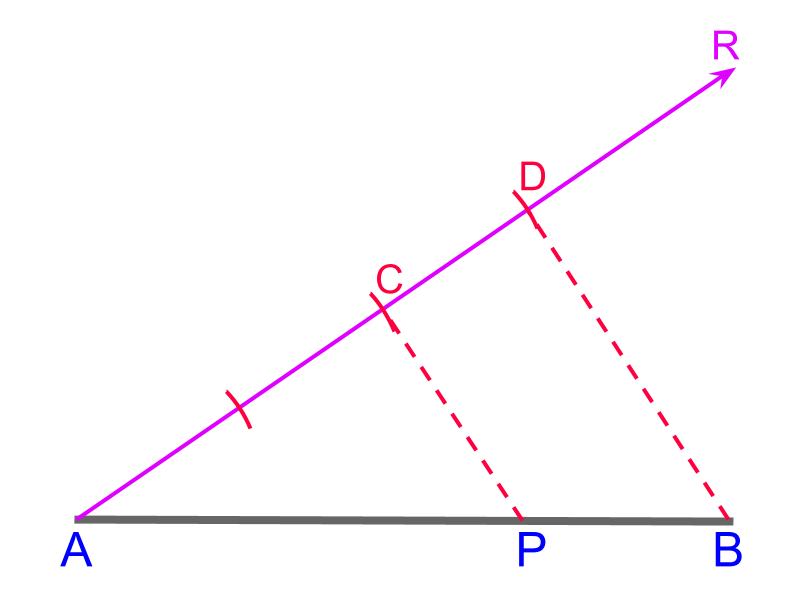Continuing on dividing line segment $\overline{AB}$$\overline{A B}$ in ratio $\overline{AP}:\overline{PB}=2:1$$\overline{A P} : \overline{P B} = 2 : 1$. The construction is visualized in the following.

•  Construct a ray $\stackrel{\to }{AR}$$\vec{A R}$ at an angle

•  In a compass, take a measurement. Any arbitrary length will do fine.

•  Using the compass, mark a number of segments on the ray.

•  Construct line $D$$D$ to $B$$B$

•  Construct a line through the the point $C$$C$

•  The point of intersection of line through $C$$C$ and $\overline{AB}$$\overline{A B}$ is marked $P$$P$.Continuing on dividing $\overline{AB}$$\overline{A B}$ in ratio $2:1$$2 : 1$. The figure illustrates $3$$3$ different measures on compass. The procedure to construct will work for any arbitrary length.Continuing on dividing line segment $\overline{AB}$$\overline{A B}$ in ratio $\overline{AP}:\overline{PB}=2:1$$\overline{A P} : \overline{P B} = 2 : 1$. The construction is visualized in the following.

•  Construct a ray $\stackrel{\to }{AR}$$\vec{A R}$ at an angle

•  In a compass, take a measurement

•  Using the compass, mark a number of segments on the ray. To achieve $2:1$$2 : 1$, sum of $2$$2$ and $1$$1$ = $3$$3$ segments are marked.,

•  Construct line $D$$D$ to $B$$B$

•  Construct a parallel to $\overline{DB}$$\overline{D B}$ through the the point $C$$C$

•  The point of intersection of parallel through $C$$C$ and $\overline{AB}$$\overline{A B}$ is marked $P$$P$.Continuing on dividing $\overline{AB}$$\overline{A B}$ in ratio $2:1$$2 : 1$. The lines through points $C1$$C 1$, $C2$$C 2$, and $C3$$C 3$ are parallel to $\overline{D1B}$$\overline{D 1 B}$, $\overline{D2B}$$\overline{D 2 B}$, and $\overline{D3B}$$\overline{D 3 B}$ respectively. By the rules of similar triangles, the lines intersect at point $P$$P$.The construction is visualized in the following.

•  Construct a ray $\stackrel{\to }{AR}$$\vec{A R}$ at an angle

•  In a compass, take a measurement

•  Using the compass, mark a number of segments on the ray.

•  Construct line $D$$D$ to $B$$B$

•  Construct a parallel to $\overline{DB}$$\overline{D B}$ through the point $C$$C$

•  The point of intersection of the parallel through $C$$C$ and $\overline{AB}$$\overline{A B}$ is marked $P$$P$.

The ratio $\overline{AP}:\overline{PB}$$\overline{A P} : \overline{P B}$ is same as the ratio $\overline{AC}:\overline{CD}$$\overline{A C} : \overline{C D}$, which is $2:1$$2 : 1$

summarySegmenting a line in a given ratio (Triangle Method): Use the property of similar triangles the given line can be segmented. Given $\overline{AB}$$\overline{A B}$ to be divided in ratio $m:n$$m : n$.

The triangle $△ABD$$\triangle A B D$ is constructed with $\overline{AD}$$\overline{A D}$ having $m+n$$m + n$ segments. By using the first $m$$m$ segments, the similar triangle $△ACP$$\triangle A C P$ is created.

Since, $\overline{AC}:\overline{CD}$$\overline{A C} : \overline{C D}$ is $m:n$$m : n$, by the similarity property of triangle, $\overline{AP}:\overline{PB}=m:n$$\overline{A P} : \overline{P B} = m : n$

segmenting a line

The objective is to divide the given line $\overline{AB}$$\overline{A B}$ in ratio $m:n$$m : n$. The properties of similar triangles help in acheiving that.A set of similar triangles are formed with $\overline{AB}$$\overline{A B}$ and a pair of parallel lines. The formulation is shown in the figure. In such a construction, the following properties are noted.

•  $\overline{AD}\mid \mid \overline{BC}$$\overline{A D} | | \overline{B C}$

•  $\overline{AP}:\overline{AE}=\overline{PB}:\overline{BF}$$\overline{A P} : \overline{A E} = \overline{P B} : \overline{B F}$

The construction is explained in the following pages.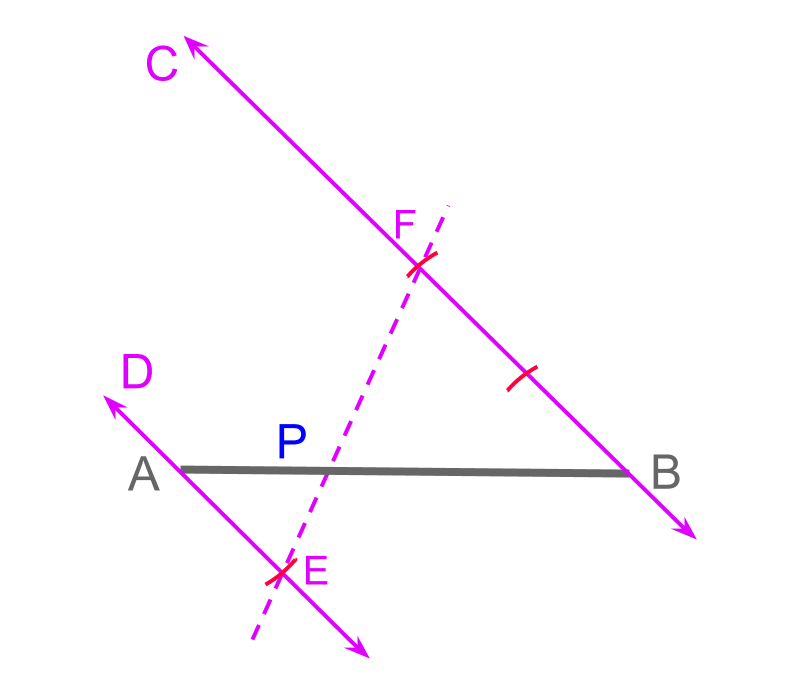The objective is to divide the given line $\overline{AB}$$\overline{A B}$ in ratio $\overline{AP}:\overline{PB}=1:2$$\overline{A P} : \overline{P B} = 1 : 2$.

The construction is visualized in the following.

•  Construct a ray $\stackrel{\to }{BC}$$\vec{B C}$ at an angle. Any angles will do fine.

•  Construct another ray $\stackrel{\to }{AD}$$\vec{A D}$ in parallel to $\stackrel{\to }{BC}$$\vec{B C}$.

•  In a compass, take a measurement. Any arbitrary length will do fine.

•  Using the compass, mark a number of segments on the rays $\stackrel{\to }{AD}$$\vec{A D}$ and $\stackrel{\to }{BC}$$\vec{B C}$ marking points $E$$E$ and $F$$F$.

•  Construct line $E$$E$ to $F$$F$

•  The point $P$$P$ is marked at the intersection of $\overline{EF}$$\overline{E F}$ and $\overline{AB}$$\overline{A B}$.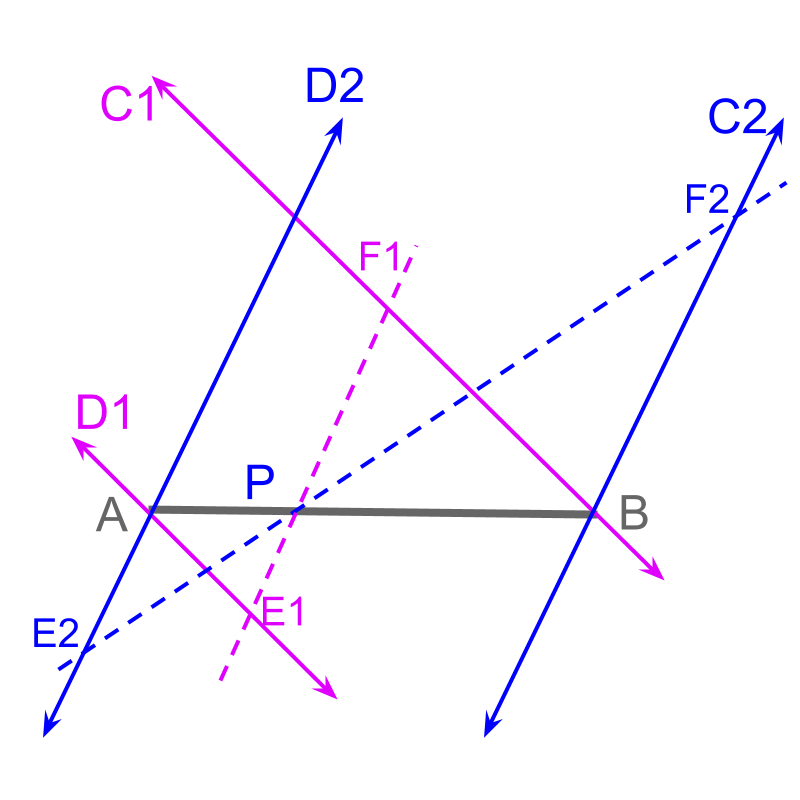The figure illustrates $3$$3$ different angles. The procedure will work for any arbitrary measure of angle.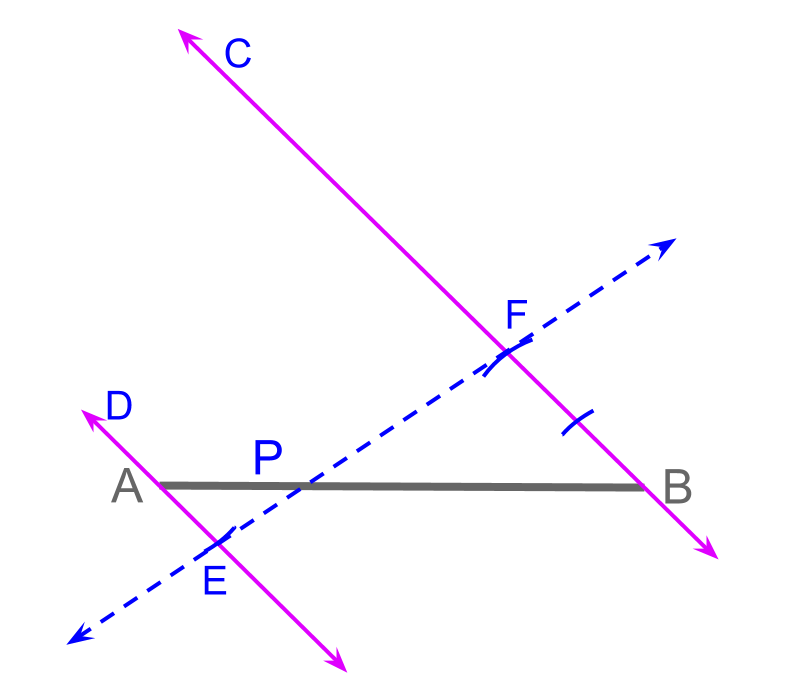The construction is visualized as given below.

•  Construct a ray $\stackrel{\to }{BC}$$\vec{B C}$ at an angle

•  Construct another ray $\stackrel{\to }{AD}$$\vec{A D}$ in parallel to the $\stackrel{\to }{BC}$$\vec{B C}$.

•  In a compass, take an arbitrary length on the compass

•  Using the compass, mark a number of segments on the rays $\stackrel{\to }{AD}$$\vec{A D}$ and $\stackrel{\to }{BC}$$\vec{B C}$ marking points $E$$E$ and $F$$F$.

•  Construct line $E$$E$ to $F$$F$

•  The point $P$$P$ is marked at the intersection of $\overline{EF}$$\overline{E F}$ and $\overline{AB}$$\overline{A B}$.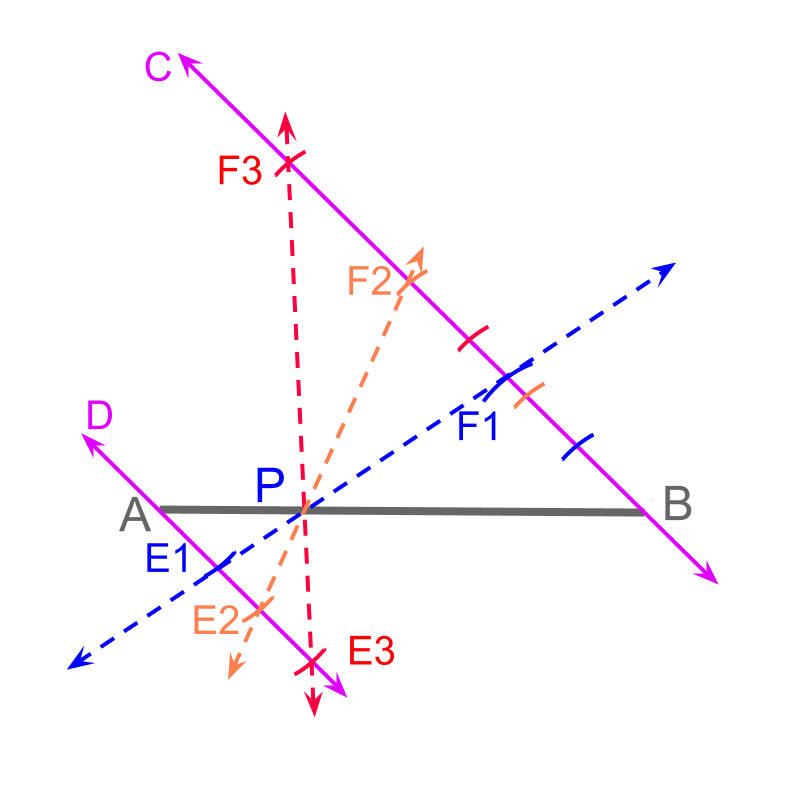The figure illustrates $3$$3$ different measures on compass $\overline{AE1}$$\overline{A E 1}$, $\overline{AE2}$$\overline{A E 2}$, $\overline{AE3}$$\overline{A E 3}$. The procedure to construct will work for any arbitrary length.Continuing on dividing $\overline{AB}$$\overline{A B}$ in ratio $\overline{AP}:\overline{PB}=1:2$$\overline{A P} : \overline{P B} = 1 : 2$.

•  Using the measure on the compass, mark a number of segments on the rays $\stackrel{\to }{AD}$$\vec{A D}$ and $\stackrel{\to }{BC}$$\vec{B C}$ marking points $E$$E$ and $F$$F$. To achieve $1:2$$1 : 2$ ratio, the points are marked with $1$$1$ segment on $\stackrel{\to }{AD}$$\vec{A D}$ and $2$$2$ segments on $\stackrel{\to }{BC}$$\vec{B C}$

From the properties of similar trianges, $\overline{AP}:\overline{PB}=1:2$$\overline{A P} : \overline{P B} = 1 : 2$, same as the ratio $\overline{AE}:\overline{BF}$$\overline{A E} : \overline{B F}$.

summarySegmenting a line in a given Ratio (Traversal of pair of parallels) : Use the properties of angles in the traversal of parallel lines and the similar triangles, the given line can be segmented. Given $\overline{AB}$$\overline{A B}$ to be divided in ratio $m:n$$m : n$.

The parallels $\stackrel{\to }{AD}$$\vec{A D}$ and $\stackrel{\to }{BC}$$\vec{B C}$ are constructed.

Mark points $E$$E$ and $F$$F$ in the given ratio $m:n$$m : n$. Since $\overline{AE}:\overline{BF}$$\overline{A E} : \overline{B F}$ is in the given ratio $m:n$$m : n$, by the similarity property of triangles, $\overline{AP}:\overline{PB}=m:n$$\overline{A P} : \overline{P B} = m : n$

scale-down a triangle

Consider the given $△ABC$$\triangle A B C$. The objective is to construct $△APQ$$\triangle A P Q$ such that the corresponding sides are in ratio $3:2$$3 : 2$. To achieve that, the first step is to divide the side $\overline{AB}$$\overline{A B}$ to mark point $P$$P$. Then $\overline{PQ}$$\overline{P Q}$ can be constructed to complete $△APQ$$\triangle A P Q$.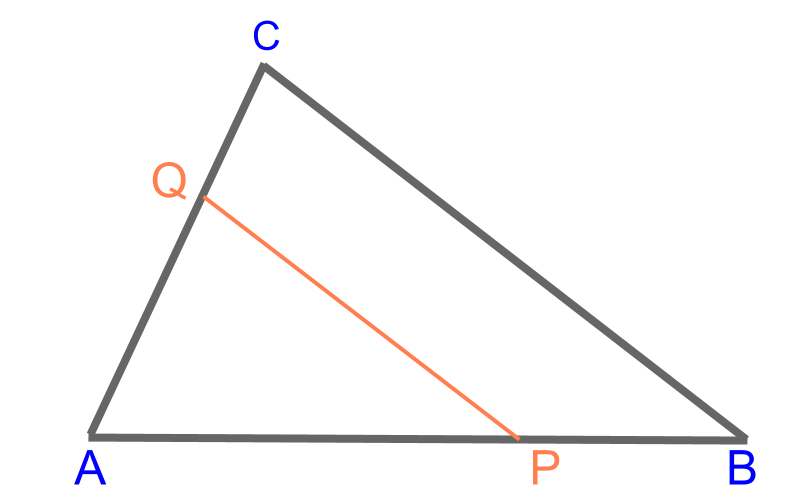Considering scaling the given $△ABC$$\triangle A B C$ to $△APQ$$\triangle A P Q$ in the ratio $3:2$$3 : 2$. The given ratio is for the corresponding sides of the triangles, i.e. $\overline{AB}:\overline{AP}$$\overline{A B} : \overline{A P}$. But to divide the $\overline{AB}$$\overline{A B}$, the ratio is modified to $\overline{AP}:\overline{PB}=2:1$$\overline{A P} : \overline{P B} = 2 : 1$.Considering scaling the given $△ABC$$\triangle A B C$ to $△APQ$$\triangle A P Q$ in the ratio $3:2$$3 : 2$. The figure illustrates the solution. The $\overline{AB}$$\overline{A B}$ is segmented to $\overline{AP}$$\overline{A P}$ and $\overline{PB}$$\overline{P B}$. This marks the point $P$$P$.

To mark $Q$$Q$, construct the line $\overline{PQ}$$\overline{P Q}$ in parallel to $\overline{BC}$$\overline{B C}$.

summaryScaling a Triangle:The figure illustrates the solution. The $\overline{AB}$$\overline{A B}$ is segmented to $\overline{AP}$$\overline{A P}$ and $\overline{PB}$$\overline{P B}$. This marks the point $P$$P$.

The $\overline{PQ}$$\overline{P Q}$ is constructed in parallel to $\overline{BC}$$\overline{B C}$.

$△APQ$$\triangle A P Q$ is constructed.

scale-up a triangle

Consider the given $△ABC$$\triangle A B C$. The objective is to construct $△APQ$$\triangle A P Q$ such that the corresponding sides are in the ratio $2:3$$2 : 3$. To achieve that, one of the sides can be scaled-up to construct similar triangles.

Scale-up the side $\overline{AB}$$\overline{A B}$ to mark point $P$$P$;. Then $\overline{PQ}$$\overline{P Q}$ can be constructed to complete $△APQ$$\triangle A P Q$.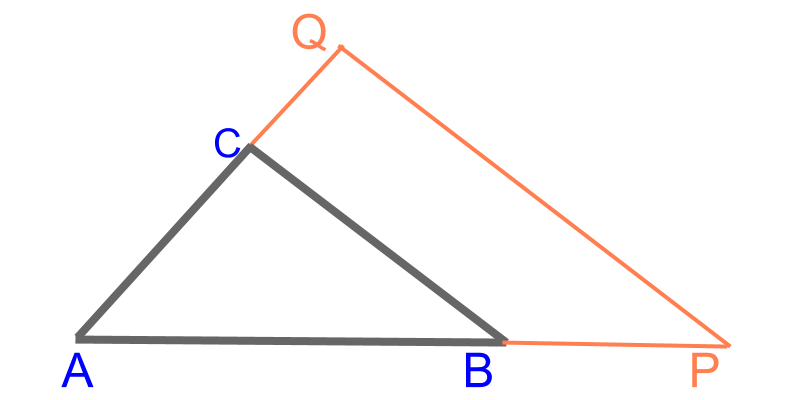Considering scaling the given $△ABC$$\triangle A B C$ to $△APQ$$\triangle A P Q$ in the ratio $2:3$$2 : 3$. The given ratio is for the corresponding sides of the triangles, i.e. $\overline{AB}:\overline{AP}$$\overline{A B} : \overline{A P}$. But to scale the $\overline{AB}$$\overline{A B}$, the ratio is modified to $\overline{AB}:\overline{BP}=2:1$$\overline{A B} : \overline{B P} = 2 : 1$.The figure illustrates the solution. The $\overline{AB}$$\overline{A B}$ is scaled to $\overline{AB}$$\overline{A B}$ and $\overline{BP}$$\overline{B P}$. This marks the point $P$$P$. To mark $Q$$Q$, construct the line $\overline{PQ}$$\overline{P Q}$ in parallel to $\overline{BC}$$\overline{B C}$ and extend $\overline{AC}$$\overline{A C}$.

The figure illustrates the solution. The $\overline{AB}$$\overline{A B}$ is scaled up to $\overline{AB}$$\overline{A B}$ and $\overline{BP}$$\overline{B P}$. This marks the point $P$$P$.

The $\overline{AC}$$\overline{A C}$ is extended to the ray $\stackrel{\to }{AT}$$\vec{A T}$. The $\overline{PQ}$$\overline{P Q}$ is constructed in parallel to $\overline{BC}$$\overline{B C}$ and that meets $\stackrel{\to }{AT}$$\vec{A T}$.

$△APQ$$\triangle A P Q$ is constructed.

summaryScaling of Triangles : To scale a triangle, scale one of the sides and use the properties of similar triangles to construct the scaled triangle.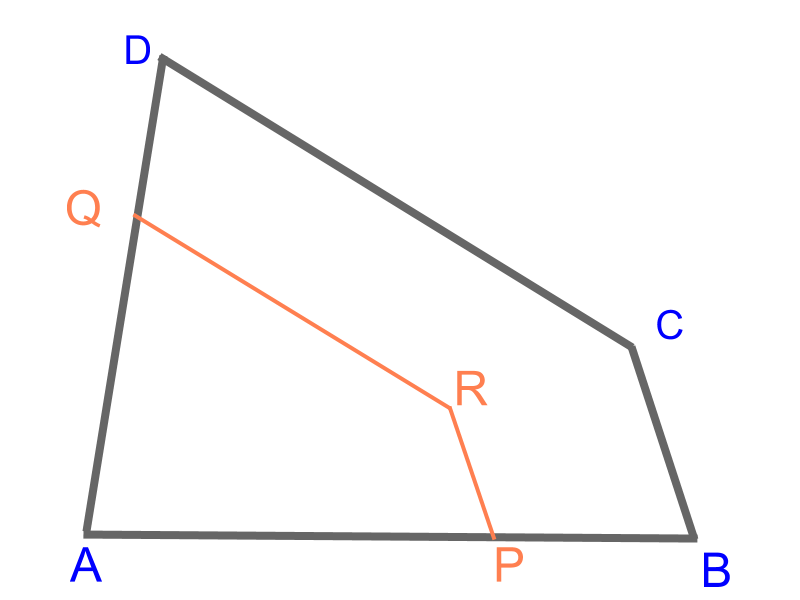Consider the given quadrilateral $ABCD$$A B C D$. The objective is to construct the quadrilateral $APRQ$$A P R Q$ such that the corresponding sides are in ratio $3:2$$3 : 2$. To achieve that, divide the sides, to mark points $P$$P$ and $Q$$Q$ and construct parallels to mark point $R$$R$.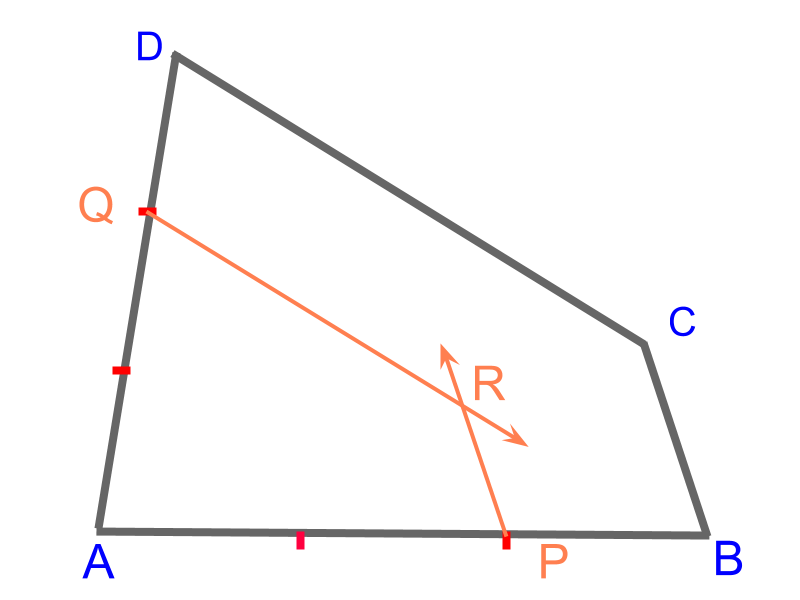Consider the given quadrilateral $ABCD$$A B C D$. The objective is to construct the quadrilateral $APRQ$$A P R Q$ such that the corresponding sides are in ratio $3:2$$3 : 2$.

The following steps details construction of the quadrilateral $APRQ$$A P R Q$.

•  Divide the side $AB$$A B$ to $2:1$$2 : 1$ ratio to mark point $P$$P$.

•  Divide the side $AD$$A D$ to $2:1$$2 : 1$ ratio to mark point $Q$$Q$.

•  Construct two rays $\stackrel{\to }{PR}$$\vec{P R}$ and $\stackrel{\to }{QR}$$\vec{Q R}$ in parallel to $\overline{BC}$$\overline{B C}$ and $\overline{DC}$$\overline{D C}$ respectively.

•  The point of intersection is point $R$$R$.

Quadrilateral $APRQ$$A P R Q$ is constructed.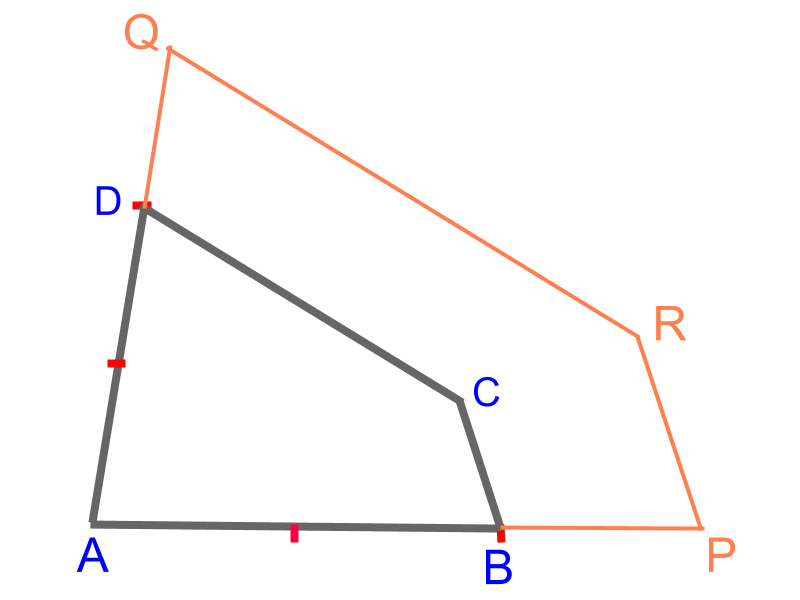Consider the given quadrilateral $ABCD$$A B C D$. The objective is to construct the quadrilateral $APRQ$$A P R Q$ such that the corresponding sides are in ratio $2:3$$2 : 3$. To achieve that scale-up the sides, to mark points $P$$P$ and $Q$$Q$ and construct parallels to mark point $R$$R$Consider the given quadrilateral $ABCD$$A B C D$. The objective is to construct the quadrilateral $APRQ$$A P R Q$ such that the corresponding sides are in ratio $2:3$$2 : 3$

The following steps details construction of the quadrilateral $APRQ$$A P R Q$.

•  Scale-up the side $AB$$A B$ to $2:1$$2 : 1$ ratio to mark point $P$$P$.

•  Scale-up the side $AD$$A D$ to $2:1$$2 : 1$ ratio to mark point $Q$$Q$.

•  Construct two rays $\stackrel{\to }{PR}$$\vec{P R}$ and $\stackrel{\to }{QR}$$\vec{Q R}$ in parallel to $\overline{BC}$$\overline{B C}$ and $\overline{DC}$$\overline{D C}$ respectively.

•  The point of intersection is point $R$$R$

Quadrilateral $APRQ$$A P R Q$ is constructed.

summaryScaling of Polygons : To scale a polygon, scale a side and construct a parallel to the next side and scale the side in the parallel. Continue this with all the sides to complete scaling the given polygon.

scaling a circle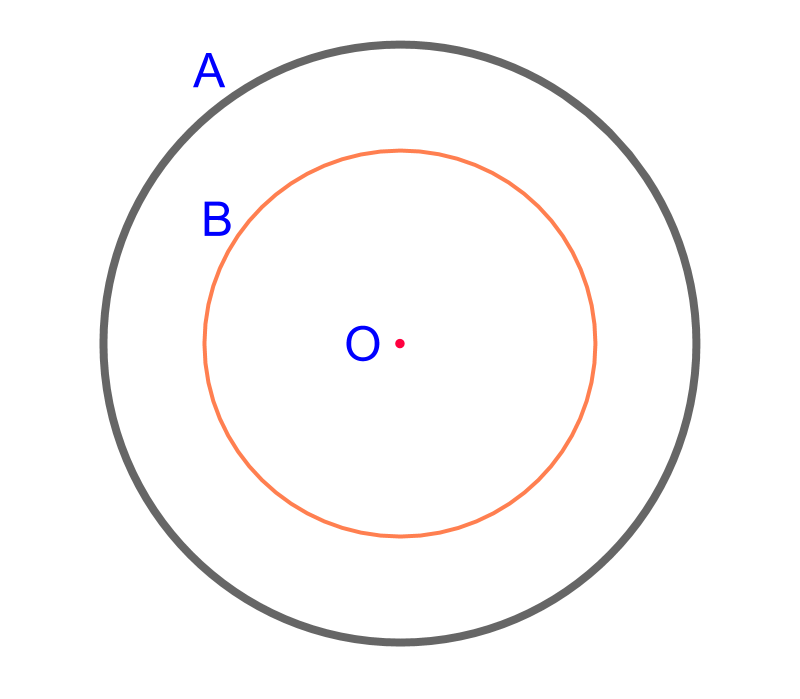Consider the given circle $A$$A$ centered at point $O$$O$. The objective is to construct circle $B$$B$ such that the radius are in ratio $3:2$$3 : 2$. To achieve that, construct a radius and divide it in $2:1$$2 : 1$ ratioConsider the given circle $A$$A$ centered at point $O$$O$. The objective is to construct circle $B$$B$ such that the radius are in ratio $3:2$$3 : 2$

The following steps detail scaling a circle.

•  Construct a radius $\overline{OP}$$\overline{O P}$.

•  Scale the radius in ratio $2:1$$2 : 1$ to mark point $Q$$Q$.

•  Construct a circle with radius $\overline{OQ}$$\overline{O Q}$. Scaled Circle $B$$B$ is constructed.

summaryScaling a Circle : To scale a circle, scale a radius as per scaling a line segment. The scaled circle is constructed with scaled line segment as the radius.

Outline

The outline of material to learn "Consrtruction (High school)" is as follows.